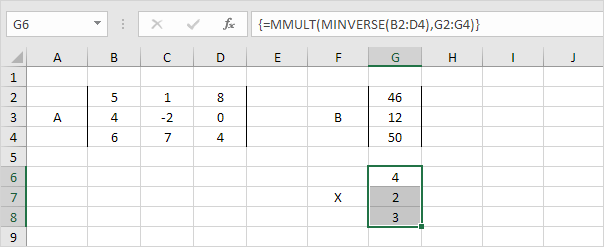# System of Linear Equations

This example shows you how to solve a system of linear equations in Excel. For example, we have the following system of linear equations:

 5x + 1y + 8z = 46 4x – 2y = 12 6x + 7y + 4z = 50

In matrix notation, this can be written as AX = B

 5 1 8 x 46 with A = 4 -2 0 , X = y , B = 12 6 7 4 z 50

If A-1 (the inverse of A) exists, we can multiply both sides by A-1 to obtain X = A-1B. To solve this system of linear equations in Excel, execute the following steps.

1. Use the MINVERSE function to return the inverse matrix of A. First, select the range B6:D8. Next, insert the MINVERSE function shown below. Finish by pressing CTRL + SHIFT + ENTER.Note: the formula bar indicates that the cells contain an array formula. Therefore, you cannot delete a single result. To delete the results, select the range B6:D8 and press Delete.

2. Use the MMULT function to return the product of matrix A-1 and B. First, select the range G6:G8. Next, insert the MMULT function shown below. Finish by pressing CTRL + SHIFT + ENTER.3. Put it all together. First, select the range G6:G8. Next, insert the formula shown below. Finish by pressing CTRL + SHIFT + ENTER.Previous articleShade Alternate Rows in Excel
Next articleTax Rates in Excel